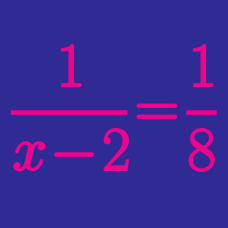Algebra

# Algebraic Expressions - Fractions

Which of the following is the simplified expression of

$\frac{x^2 - 3x + 2}{x^2 - x - 6} \times \frac{x^2 - 4x + 3}{x^2 - 4}?$

Simplify

$\frac{1}{x (x+2)} + \frac{1}{(x+2) (x+4)} + \frac{1}{(x+4) (x+6)} + \frac{1}{(x+6) (x+8)}.$

Simplify

$1 - \frac{1}{1 - \dfrac{1}{1-x}}.$

If real numbers $$x$$ and $$y$$ satisfy $$\frac{x^3+y^3}{x^3-y^3} = \frac{7}{9},$$ what is the value of $\frac{x+y}{x-y}?$

Which of the following is the simplified expression of

$\frac{2r}{r^2 - s^2} + \frac{1}{r+s} - \frac{1}{r-s} ?$

×